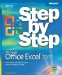# Creating Formulas More Easily by Using Formula AutoComplete

 Excel 2003 and earlier versions of the program provided two methods to find the name of a function to add to a formula: the help system and the Insert Function dialog box. Excel 2007 adds a new tool to your arsenal: Formula AutoComplete. Here's how it works: When you begin typing a formula into a cell, Excel 2007 examines what you're typing and then displays a list of functions and function arguments, such as named cell ranges or table columns that could be used in the formula. Formula AutoComplete offers lists of the following items as you create a formula: Excel 2007 functions. Typing the characters =SUB into a cell causes Excel 2007 to display a list with the functions SUBSTITUTE and SUBTOTAL. Clicking the desired function name adds that function to your formula without requiring you to finish typing the function's name.User-defined functions. User-defined functions are custom procedures created by a programmer and are included in your workbook as macro code.Formula arguments. Some formulas accept a limited set of values for a function argument; Formula AutoComplete enables Excel 2007 to select from the list of acceptable values.Defined names. Defined names are user-defined nicknames for specific cell ranges (for example, cells A2:A29 on the Sales worksheet could be named February Sales).Table structure references. A table structure reference denotes a table or part of a table. For example, if you create a table named Package Volume that contains a column named Northwest, typing the letter P in a formula prompts Excel 2007 to display the table name Package Volume as a possible entry into the formula.MicrosoftВ® Office ExcelВ® 2007 Step by Step (Step By Step (Microsoft))
ISBN: 073562304X
EAN: 2147483647
Year: 2004
Pages: 143
Authors: Curtis Frye

Similar book on Amazon